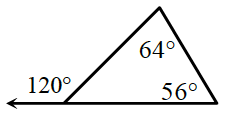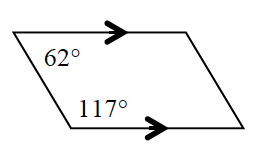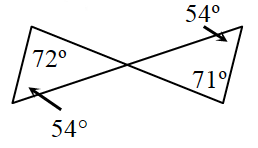### Home > CCG > Chapter 9 > Lesson 9.1.5 > Problem9-62

9-62.

Examine the information provided in each diagram below. Decide if each figure is possible or not. If the figure is not possible, explain why.1.What angle measure is supplementary to $120^\circ$?
Would this also satisfy the Triangle Angle Sum Theorem?

1.How are the same-side interior angles in a parallelogram related?

1.Are the vertical angles equal?